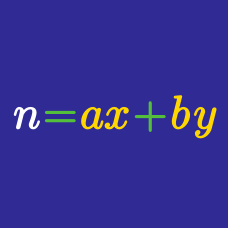Number Theory

# Integer Equations - With Restriction

Ben throws a fair die five times. If we label the outcome of the $n^{\text{th}}$ throw as $a_n,$ what is the number of cases such that $a_1< a_2

Consider the two sets $A=\{1,2,3,4,5,6\}$ and $B=\{7,8,9,10\}.$ How many functions $f:A\rightarrow B$ are there that satisfy $f(1)\le f(2)\le f(3)

How many 4-digit numbers are there such that the digit sum is $9?$

How many 4-digit numbers are there such that the digit sum is $11?$

Ben throws a fair die six times. If we label the outcome of the $n^{\text{th}}$ throw as $a_n,$ what is the number of cases such that $a_1\le a_2

×